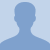# Konst Math: Adding and Subtracting DecimalsAdding and subtracting decimals is very similar to performing the same with any whole numbers. If you know how to add and subtract whole numbers, then you can add and subtract decimals! Just be sure to... Adding and subtracting decimals is very similar to performing the same with any whole numbers. If you know how to add and subtract whole numbers, then you can add and subtract decimals! Just be sure to line up the terms so that all the decimal points are in a vertical line.
More... Collapse
111 Views

## Comments (0)

Please log in to post comments.